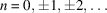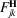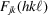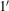International Tables for Crystallography (2013). Vol. D, ch. 1.11, pp. 269-283https://doi.org/10.1107/97809553602060000910

## Contents

• 1.11. Tensorial properties of local crystal susceptibilities  (pp. 269-283)
• 1.11.1. Introduction  (pp. 269-270) | html | pdf |
• 1.11.2. Symmetry restrictions on local tensorial susceptibility and forbidden reflections  (pp. 270-272) | html | pdf |
• 1.11.2.1. General symmetry restrictions  (p. 270) | html | pdf |
• 1.11.2.2. Tensorial structure factors and forbidden reflections  (pp. 270-271) | html | pdf |
• 1.11.2.2.1. Glide-plane forbidden reflections  (pp. 270-271) | html | pdf |
• 1.11.2.2.2. Screw-axis forbidden reflections  (p. 271) | html | pdf |
• 1.11.2.3. Local tensorial susceptibility of cubic crystals  (pp. 271-272) | html | pdf |
• 1.11.3. Polarization properties and azimuthal dependence  (pp. 272-274) | html | pdf |
• 1.11.4. Physical mechanisms for the anisotropy of atomic X-ray susceptibility  (p. 274) | html | pdf |
• 1.11.5. Non-resonant magnetic scattering  (p. 275) | html | pdf |
• 1.11.6. Resonant atomic factors: multipole expansion  (pp. 275-280) | html | pdf |
• 1.11.6.1. Tensor atomic factors: internal symmetry  (pp. 275-276) | html | pdf |
• 1.11.6.2. Tensor atomic factors (non-magnetic case)  (pp. 276-277) | html | pdf |
• 1.11.6.3. Hidden internal symmetry of the dipole–quadrupole tensors in resonant atomic factors  (pp. 277-278) | html | pdf |
• 1.11.6.4. Tensor structure factors  (p. 278) | html | pdf |
• 1.11.6.5. Tensor atomic factors (magnetic case)  (pp. 278-279) | html | pdf |
• 1.11.6.6. Tensor atomic factors (spherical tensor representation)  (pp. 279-280) | html | pdf |
• 1.11.7. Glossary  (p. 281) | html | pdf |
• References | html | pdf |
• Tables
• Table 1.11.2.1. The indicesof the screw-axis/glide-plane forbidden reflections () and independent components of their tensorial structure factors(p. 271) | html | pdf |
• Table 1.11.2.2. The indices of the forbidden reflections and corresponding tensors of structure factorsfor the cubic space groups ()  (p. 272) | html | pdf |
• Table 1.11.6.1. Coefficientscorresponding to various kinds of tensor symmetry with respect to space inversion, rotations, and time reversal(p. 275) | html | pdf |
• Table 1.11.6.2. Identification of properties under time inversionand space inversionof tensors associated with multipole expansion  (p. 280) | html | pdf |